# Fractions

###### Question 1.Write the fraction representing the shaded portion: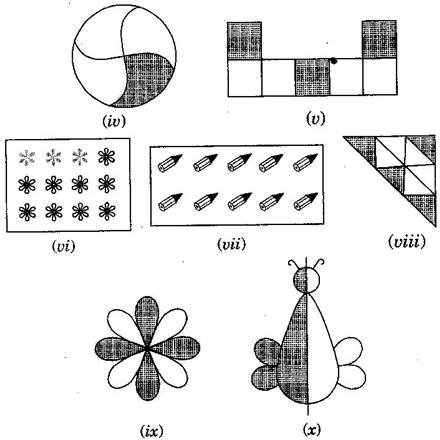(i)2424

(ii)8989

(iii)4848

(iv)1414

(v)3737

(vi)312312

(vii)10101010

(viii)4949

(ix)4848

(x)1212

###### Question 2.Colour the part according to the given fraction: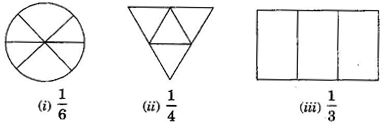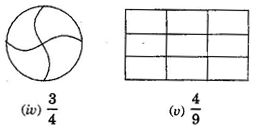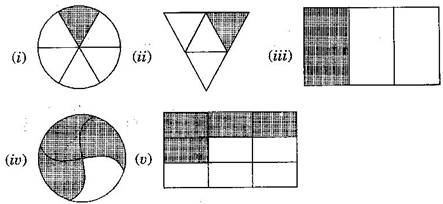NCERT Solutions for Class 6 Maths Exercise 7.1

###### Question 3.Identify the error, if any?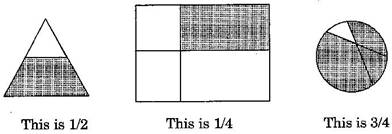All the figures are not equally divided. For making fractions, it is necessary that figure is to be divided in equal parts.

###### Question 4.What fraction of a day is 8 hours?

Since, 1 day = 24 hours.

Therefore, the fraction of 8 hours = 824=13824=13

NCERT Solutions for Class 6 Maths Exercise 7.1

###### Question 5.What fraction of an hour is 40 minutes?

Since, 1 hour = 60 minutes.

Therefore, the fraction of 40 minutes = 4060=234060=23

###### Question 6.Arya, Abhimanyu and Vivek shared lunch. Arya has brought two sandwiches, one made of vegetable and one of jam. The other two boys forgot to bring their lunch. Arya agreed to share his sandwiches so that each person will have an equal share of each sandwich.

(a)How can Arya divide his sandwiches so that each person has an equal share?

(b)What part of a sandwich will each boy receive?

(a) Arya will divide each sandwich into three equal parts and give one part of each sandwich to

each one of them.

(b) 1×13=131×13=13

NCERT Solutions for Class 6 Maths Exercise 7.1

###### Question 7.Kanchan dyes dresses. She had to dye 30 dresses. She has so far finished 20 dresses. What fraction of dresses has she finished?

Total number of dresses = 30

Work finished = 20

Fraction of finished work = 2030=232030=23

###### Question 8.Write the natural numbers from 2 to 12. What fraction of them are prime numbers?

Natural numbers from 2 to 12: 2, 3, 4, 5, 6, 7, 8, 9, 10, 11, 12

Prime numbers from 2 to 12: 2, 3, 5, 7, 11

Hence, fraction of prime numbers = 511511

NCERT Solutions for Class 6 Maths Exercise 7.1

###### Question 9.Write the natural numbers from 102 to 113. What fraction of them are prime numbers?

Natural numbers from 102 to 113: 102, 103, 104, 105, 106, 107, 108, 109, 110, 111, 112, 113

Prime numbers from 102 to 113: 103, 107, 109, 113

Hence fraction of prime numbers = 412=13412=13

###### Question 10.What fraction of these circles have ‘X’s in them?Total number of circles = 8 and number of circles having ‘X’ = 4

Hence, the fraction = 4848

NCERT Solutions for Class 6 Maths Exercise 7.1

###### Question 11.Kristin received a CD player for her birthday. She bought 3 CDs and received 5 others as gifts. What fraction of her total CDs did she buy and what fraction did she receive as gifts?

Total number of CDs = 3 + 5 = 8

Number of CDs purchased = 3

Fraction of CDs purchased = 3838

###### Question 1.Draw number lines and locate the points on them:

(a)12,14,34,4412,14,34,44

(b)18,28,38,7818,28,38,78

(c)25,35,85,4525,35,85,45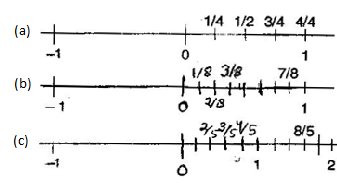NCERT Solutions for Class 6 Maths Exercise 7.2

###### Question 2.Express the following fractions as mixed fractions:

(a)203203

(b)115115

(c)177177

(d)285285

(e)196196

(f)359359

(a)

3{tex}\mathop{\left){\vphantom{1\begin{gathered}

{\text{ }}20 \\\\\\

\underline { – 18} \\\\\\

\underline {{\text{ 2}}} \\\\\\

\end{gathered} }}\right.

\!\!\!\!\overline{\,\,\,\vphantom 1{\begin{gathered}

{\text{ }}20 \\\\\\

\underline { – 18} \\\\\\

\underline {{\text{ 2}}} \\\\\\

\end{gathered} }}}

\limits^{\displaystyle\,\,\, 6}{/tex}

(b)

5{tex}\mathop{\left){\vphantom{1\begin{gathered}

{\text{ 11}} \\\\\\

\underline { – 10} \\\\\\

\underline {{\text{ 1}}} \\\\\\

\end{gathered} }}\right.

\!\!\!\!\overline{\,\,\,\vphantom 1{\begin{gathered}

{\text{ 11}} \\\\\\

\underline { – 10} \\\\\\

\underline {{\text{ 1}}} \\\\\\

\end{gathered} }}}

\limits^{\displaystyle\,\,\, 2}{/tex}

203=623203=623

115=215115=215

(c)

7{tex}\mathop{\left){\vphantom{1\begin{gathered}

{\text{ 17}} \\\\\\

\underline { – 14} \\\\\\

\underline {{\text{ 3}}} \\\\\\

\end{gathered} }}\right.

\!\!\!\!\overline{\,\,\,\vphantom 1{\begin{gathered}

{\text{ 17}} \\\\\\

\underline { – 14} \\\\\\

\underline {{\text{ 3}}} \\\\\\

\end{gathered} }}}

\limits^{\displaystyle\,\,\, 2}{/tex}

(d)

5{tex}\mathop{\left){\vphantom{1\begin{gathered}

{\text{ 28}} \\\\\\

\underline { – 25} \\\\\\

\underline {{\text{ 3}}} \\\\\\

\end{gathered} }}\right.

\!\!\!\!\overline{\,\,\,\vphantom 1{\begin{gathered}

{\text{ 28}} \\\\\\

\underline { – 25} \\\\\\

\underline {{\text{ 3}}} \\\\\\

\end{gathered} }}}

\limits^{\displaystyle\,\,\, 5}{/tex}

177=237177=237

285=535285=535

(e)

6{tex}\mathop{\left){\vphantom{1\begin{gathered}

{\text{ 19}} \\\\\\

\underline { – 18} \\\\\\

\underline {{\text{ 1}}} \\\\\\

\end{gathered} }}\right.

\!\!\!\!\overline{\,\,\,\vphantom 1{\begin{gathered}

{\text{ 19}} \\\\\\

\underline { – 18} \\\\\\

\underline {{\text{ 1}}} \\\\\\

\end{gathered} }}}

\limits^{\displaystyle\,\,\, 3}{/tex}

(f)

9{tex}\mathop{\left){\vphantom{1\begin{gathered}

{\text{ 35}} \\\\\\

\underline { – 27} \\\\\\

\underline {{\text{ 8}}} \\\\\\

\end{gathered} }}\right.

\!\!\!\!\overline{\,\,\,\vphantom 1{\begin{gathered}

{\text{ 35}} \\\\\\

\underline { – 27} \\\\\\

\underline {{\text{ 8}}} \\\\\\

\end{gathered} }}}

\limits^{\displaystyle\,\,\, 3}{/tex}

196=316196=316

359=389359=389

NCERT Solutions for Class 6 Maths Exercise 7.2

###### Question 3.Express the following as improper fractions:

(a)734734

(b)567567

(c)256256

(d)10351035

(e)937937

(f)849849

(a)734=(7×4)+34=28+34=314734=(7×4)+34=28+34=314

(b)567=(5×7)+67=35+67=417567=(5×7)+67=35+67=417

(c)256=(2×6)+56=12+56=176256=(2×6)+56=12+56=176

(d)1035=(10×5)+35=50+35=5351035=(10×5)+35=50+35=535

(e)937=(9×7)+37=63+37=667937=(9×7)+37=63+37=667

(f)849=(8×9)+49=72+49=769

###### Question 1.Write the fractions. Are all these fractions equivalent:

(a)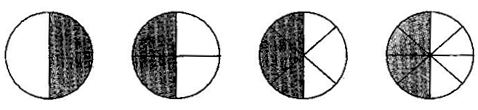(b)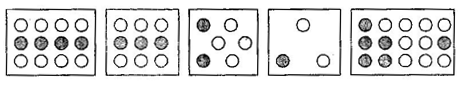(a) 12,24,36,4812,24,36,48

Yes, all of these fractions are equivalent.

(b) 412,39,26,13,615412,39,26,13,615

No, these fractions are not equivalent.

###### Question 2.Write the fraction and pair up the equivalent fractions to each row: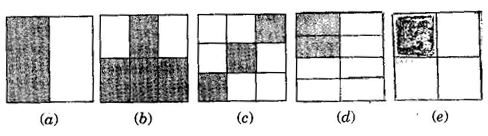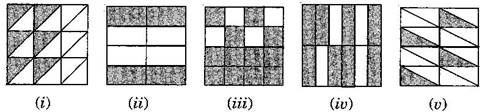(a)1212

(ii) 48=1248=12

(b) 46=2346=23

(iv) 812=23812=23

(c) 39=1339=13

(i) 618=13618=13

(d) 28=1428=14

(v) 416=14416=14

(e) 3434

(iii) 1216=341216=34

NCERT Solutions for Class 6 Maths Exercise 7.3

###### Question 3.Replace  in each of the following by the correct number:

(a) 27=8 27=8

(b) 58=10 58=10

(c) 35= 2035= 20

(d) 4560=15 4560=15

(e) 1824= 41824= 4

(a) 27=2×47×4=82827=2×47×4=828

(b) 58=5×28×2=101658=5×28×2=1016

(c) 35=3×45×4=122035=3×45×4=1220

(d) 4560=45÷360÷3=15204560=45÷360÷3=1520

(e) 1824=18÷624÷6=341824=18÷624÷6=34

###### Question 4.Find the equivalent fraction of 3535 having:

(a) denominator 20

(b) numerator 9

(c) denominator 30

(d) numerator 27

(a) 35=3×45×4=122035=3×45×4=1220

(b) 35=3×35×3=91535=3×35×3=915

(c) 35=3×65×6=183035=3×65×6=1830

(d) 35=3×95×9=274535=3×95×9=2745

NCERT Solutions for Class 6 Maths Exercise 7.3

###### Question 5.Find the equivalent fraction of 36483648 with:

(a) numerator 9

(b) denominator 4

(a) 3648=36÷448÷4=9123648=36÷448÷4=912

(b) 3648=36÷1248÷12=343648=36÷1248÷12=34

###### Question 6.Check whether the given fraction are equivalent:

(a) 59,305459,3054

(b) 310,1250310,1250

(c)713,511713,511

(a) 59,305459,3054 = 5×69×6,30545×69×6,3054 = 3054,30543054,3054

Therefore, 59,305459,3054 are equivalent.

(b) 310,1250310,1250 = 3×510×5,12503×510×5,1250 = 1550,12501550,1250

Therefore, 310,1250310,1250 are not equivalent.

(c) 713,511713,511 = 7×1113×11,5×1311×137×1113×11,5×1311×13 = 77143,6514377143,65143

Therefore, 713,511713,511 are not equivalent fraction.

NCERT Solutions for Class 6 Maths Exercise 7.3

###### Question 7.Reduce the following fractions to simplest form:

(a) 48604860

(b) 1506015060

(c) 84988498

(d) 12521252

(e) 728728

(a) 4860=2×2×2×2×32×2×3×5=454860=2×2×2×2×32×2×3×5=45

(b) 15060=3×5×102×3×10=5215060=3×5×102×3×10=52

(c) 8498=2×3×147×14=678498=2×3×147×14=67

(d) 1252=2×2×32×2×13=3131252=2×2×32×2×13=313

(e) 728=72×2×7=14728=72×2×7=14

###### Question 8.Ramesh had 20 pencils, Sheelu had 50 pencils and Jamaal had 80 pencils. After 4 months, Ramesh used up 10 pencils, Sheelu used up 25 pencils and Jamaal used up 40 pencils. What fraction did each use up? Check is each has used up an equal fraction of her/his pencils?

Ramesh: Total pencils = 20

Pencils used = 10

Fraction = 1020=121020=12

Sheelu: Total pencils = 50

Pencils used = 25

Fraction = 2550=122550=12

Jamaal: Total pencils = 80

Pencils used = 40

Fraction = 4080=124080=12

Since, all of them used half of their pencils, therefore each one used up equal fraction of pencils.

NCERT Solutions for Class 6 Maths Exercise 7.3

###### Question 9. Match the equivalent fractions and write two more for each:
 (i)250400250400 (a) 2323 (ii)180200180200 (b) 2525 (iii)660990660990 (c) 1212 (iv)180360180360 (d) 5858 (v)220550220550 (e) 910910

(i) 250400=58,1016,1524250400=58,1016,1524

(d) 5858

(ii) 180200=910,1820,2730180200=910,1820,2730

(e) 910910

(iii) 660990=23,46,69660990=23,46,69

(a) 2323

(iv) 180360=12,24,36180360=12,24,36

(c)1212

1. 220550=25,410,615220550=25,410,615

(b) 25

###### Question 1.Write shaded portion as fraction. Arrange them in ascending and descending order using correct sign ‘<’, ‘>’, ‘=’ between the fractions:

(a)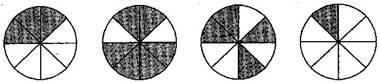(b)(c)

Show 26,46,8626,46,86 and 6666 on the number line. Put appropriate signs between the fractions given:

56 26,56 26, 36 0,36 0,

16 66,16 66, 86 5686 56

(a) 38,68,48,1838,68,48,18

Ascending order: 18<38<48<6818<38<48<68

Descending order: 68>48>38>1868>48>38>18

(b) 89,49,39,6989,49,39,69

Ascending order: 39<49<69<8939<49<69<89

Descending order: 89>69>49>3989>69>49>39

(c) Number line56>2656>26 16<6616<66

36>0636>06 86>5686>56

(a) 36 5636 56

(b) 17 1417 14

(c) 45 5545 55

(d) 35 3735 37

(a) 36<5636<56

(b) 17<1417<14

(c) 45<5545<55

(d) 35>3735>37

###### NCERT Solutions for Class 6 Maths Exercise 7.4

Question 3.Make five more each pairs and put appropriate signs.

(a) 910>610910>610

(b) 13>1613>16

(c) 18<1518<15

(d) 78<11878<118

(e) 1113>9131113>913

###### Question 4.Look at the figures and write ‘<’ or ‘>’ between the given pairs of fractions:(a) 16 1316 13

(b) 34 2634 26

(c) 23 2423 24

(d) 66 3366 33

(e) 56 5556 55

Make five more such problems and solve them with your friends.

(a) 16<1316<13

(b) 34>2634>26

(c) 23>2423>24

(d) 66=3366=33

(e) 56<5556<55

Five more such problems:

(a) 12 3612 36

(b) 23 3523 35

(c) 34 4634 46

(d) 56 2256 22

(e) 01 0601 06

Sol.

(a) 12 = 3612 = 36

(b) 23 > 3523 > 35

(c) 34 > 4634 > 46

(d) 56 < 2256 < 22

(e) 01 = 0601 = 06

NCERT Solutions for Class 6 Maths Exercise 7.4

###### Question 5.How quickly can you do this? Fill appropriate sign (<, =, >):

(a) 12 1512 15

(b) 24 3624 36

(c) 35 2335 23

(d) 34 2834 28

(e) 35 6535 65

(f) 79 3979 39

(g) 14 2814 28

(h) 610 45610 45

(i) 34 7834 78

(j) 610 45610 45

(k) 57 152157 1521

(a) 12 > 1512 > 15

(b) 24 = 3624 = 36

(c) 35 < 2335 < 23

(d) 34 > 2834 > 28

(e) 35 < 6535 < 65

(f) 79 > 3979 > 39

(g) 14 = 2814 = 28

(h) 610 < 45610 < 45

(i) 34 < 7834 < 78

(j) 610 < 45610 < 45

(k) 57 = 152157 = 1521

###### Question 6.The following fractions represent just three different numbers. Separate them into three groups of equivalent fractions, by changing each one to its simplest form:

(a) 212212

(b) 315315

(c) 850850

(d) 1610016100

(e) 10601060

(f) 15751575

(g) 12601260

(h) 16961696

(i) 12751275

(j) 12721272

(k) 318318

(l) 425425

(a) 212=16212=16

(b) 315=15315=15

(c) 850=425850=425

(d) 16100=42516100=425

(e) 1060=161060=16

(f) 1575=151575=15

(g) 1260=151260=15

(h) 1696=161696=16

(i) 1275=4251275=425

(j) 1272=161272=16

(k) 318=16318=16

(l) 425=425425=425

Equivalent groups:

I group: 1515 [(b), (f), (g)]

II group: 1616 [(a), (e), (h), (j), (k)]

III group: 425425 [(c), (d), (i), (l)]

NCERT Solutions for Class 6 Maths Exercise 7.4

###### Question 7.Find answers to the following. Write and indicate how you solved them:

(a) Is 5959 equal to 4545 ?

(b) Is 916916 equal to 5959 ?

(c) Is 4545 equal to 16201620 ?

(d) Is 115115 equal to 430430 ?

(a)5959 and 4545

5×59×5=25455×59×5=2545 and 4×95×9=36454×95×9=3645 [ L.C.M. of 9 and 5 is 45]

Since, 254536452545≠3645

Therefore, 594559≠45

(b) 916916 and 5959

9×916×9=811449×916×9=81144 and 5×169×16=801445×169×16=80144 [ L.C.M. of 16 and 9 is 144]

Since, 811448014481144≠80144

Therefore, 91659916≠59

(c) 4545 and 16201620

4×205×20=801004×205×20=80100 and 16×520×5=8010016×520×5=80100 [ L.C.M. of 5 and 20 is 100]

Since, 80100=8010080100=80100

Therefore, 45=162045=1620

(d) 115115 and 430430

1×215×2=2301×215×2=230 and 4×130×1=4304×130×1=430 [ L.C.M. of 15 and 30 is 30]

Since, 430=430430=430 Therefore,

115=430115=430

NCERT Solutions for Class 6 Maths Exercise 7.4

###### Question 8.Ila read 25 pages of a book containing 100 pages. Lalita read 2525 of the same book. Who read less?

Ila read 25 pages out of 100 pages.

Fraction of reading the pages = 25100=14th25100=14th part of book

Lalita read 25th25th part of book = 4010040100 pages

Since 14<2514<25

###### Question 9.Rafiq exercised for 3636 of an hour, while Rohit exercised for 3434 of an hour. Who exercised for a longer time?

Rafiq exercised 3636 of an hour.

Rohit exercised 3434 of an hour.

Since 34>3634>36

Therefore, Rohit exercised for a longer time.

NCERT Solutions for Class 6 Maths Exercise 7.4

###### Question 10.In a class A of 25 students, 20 passed in first class; in another class B of 30 students, 24 passed in first class. In which class was a greater fraction of students getting first class?

In class A, 20 passed out of 25, i.e., 20252025 = 4545

In class B, 24 passed out of 30, i.e., 24302430 = 4545

Hence, each class have same fraction of student getting first class.

###### Question 1.Write the fractions appropriately as additions or subtractions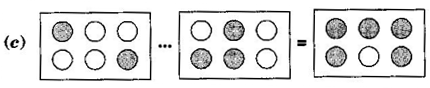(a) 15+25=1+25=3515+25=1+25=35

(b) 5535=535=2555–35=5–35=25

(c) 26+36=2+36=5626+36=2+36=56

###### Question 2.Solve:

(a) 118+118118+118

(b) 815+315815+315

(c) 775777–57

(d) 122+2122122+2122

(e) 12157151215–715

(f) 58+3858+38

(g) 123(1=33)1–23(1=33)

(h) 14+0414+04

(i) 31253–125

(a) 118+118=1+118=218=19118+118=1+118=218=19

(b) 815+315=8+315=1115815+315=8+315=1115

(c) 7757=757=2777−57=7−57=27

(d) 122+2122=1+2122=2222=1122+2122=1+2122=2222=1

(e) 1215715=12715=515=131215−715=12−715=515=13

(f) 58+38=88=158+38=88=1

(g) 123=3323=322=131−23=33−23=3−22=13

(h) 14+04=1+04=1414+04=1+04=14

(i) 3125=155125=15125=353−125=155−125=15−125=35

NCERT Solutions for Class 6 Maths Exercise 7.5

###### Question 3.Shubham painted 2323 of the wall space in his room. His sister Madhavi helped and painted 1313 of the wall space. How much did they paint together?

Fraction of wall painted by Shubham = 2323

Fraction of wall painted by Madhavi = 1313

Total painting by both of them = 23+13=2+13=33=123+13=2+13=33=1

Therefore, they painted complete wall.

###### Question 4.Fill in the missing fractions:

(a) 710 =310710– =310

(b)  321=521 –321=521

(c)  36=36 –36=36

(d)  +527=1227 +527=1227

(a) 410410

(b) 821821

(c) 6666

(d) 727727

NCERT Solutions for Class 6 Maths Exercise 7.5

###### Question 5.Javed was given of a basket of oranges. What fraction of oranges was left in the basket?

Total = 1

Fraction of Orange left = 1571–57

7757=757=2777–57=7–57=27

Thus, 2727 oranges was left in the basket.

###### Question 1.Solve:

(a) 23+1723+17

(b) 310+715310+715

(c) 49+2749+27

(d) 57+1357+13

(e) 25+1625+16

(f) 45+2345+23

(g) 341334–13

(h) 561356–13

(i) 23+34+1223+34+12

(j) 12+13+1612+13+16

(k) 113+323113+323

(l) 423+314423+314

(m) 16575165–75

(n) 431243–12

(a) L.C.M. of 3 and 7 is 21

23+17=2×7+1×321=14+321=172123+17=2×7+1×321=14+321=1721

(b) L.C.M. of 10 and 15 is 30

310+715=3×3+7×230=9+1430=2330310+715=3×3+7×230=9+1430=2330

(c) L.C.M. of 9 and 7 is 63

49+27=4×7+2×963=28+1863=466349+27=4×7+2×963=28+1863=4663

(d)L.C.M. of 7 and 3 is 21

57+13=5×3+7×121=15+721=2221=112157+13=5×3+7×121=15+721=2221=1121

(e)L.C.M. of 5 and 6 is 30

25+16=2×6+5×130=12+530=173025+16=2×6+5×130=12+530=1730

(f)L.C.M. of 5 and 3 is 15

45+2345+23 = 4×3+2×515=12+1015=2215=17154×3+2×515=12+1015=2215=1715

(g)L.C.M. of 4 and 3 is 12

3413=3×34×112=9412=51234–13=3×3–4×112=9–412=512

(h)L.C.M. of 6 and 3 is 6

5613=5×12×16=526=36=1256−13=5×1−2×16=5−26=36=12

(i)L.C.M. of 3, 4 and 2 is 12

23+34+12=2×4+3×3+1×612=6+9+612=2312=1111223+34+12=2×4+3×3+1×612=6+9+612=2312=11112

(j)L.C.M. of 2, 3, and 6 is 6

12+13+16=1×3+1×2+1×16=3+2+16=66=112+13+16=1×3+1×2+1×16=3+2+16=66=1

(k)L.C.M. of 3 and 3 is 3

43+113=4+113=153=543+113=4+113=153=5

(l)L.C.M. of 3 and 4 is 12

143+134=14×4+13×312=56+3912=9512=71112143+134=14×4+13×312=56+3912=9512=71112

(m)L.C.M. of 5 and 5 is 5

16575=1675=95=145165–75=16–75=95=145

(n)L.C.M. of 3 and 2 is 6

4312=4×21×36=836=5643–12=4×2–1×36=8–36=56

NCERT Solutions for Class 6 Maths Exercise 7.6

###### Question 2.Sarika bought 2525 meter of ribbon and Lalita 3434 meter of ribbon. What is the total length of the ribbon they bought?

Ribbon bought by Sarita = 2525 m and Ribbon bought by Lalita = 3434 m

Total length of ribbon = 25+3425+34 = 2×4+5×3202×4+5×320 [ L.C.M. of 5 and 4 is 20]

8+1520=2320=13208+1520=2320=1320 m

Therefore, they bought 13201320 m of ribbon.

NCERT Solutions for Class 6 Maths Exercise 7.6

###### Question 3.Naina was given 112112 piece of cake and Najma was given 113113 piece of cake. Find the total amount of cake given to both of them.

Cake taken by Naina = 112112 piece and Cake taken by Najma = 113113 piece

Total cake taken = 112112 + 113113 = 32+4332+43 = 3×3+4×263×3+4×26 [ L.C.M. of 2 and 3 is 6]

9+86=176=2569+86=176=256

Therefore total consumption of cake is 256256.

###### Question 4.Fill in the boxes:

(a)  58=14 –58=14

(b)  15=12 –15=12

(c) 12 =1612– =16

(a) 14+58=2+58=7814+58=2+58=78

(b) 12+15=5+210=71012+15=5+210=710

(c) 1216=316=2612–16=3–16=26

NCERT Solutions for Class 6 Maths Exercise 7.6

###### Question 5.Complete the addition – subtraction box:Sol.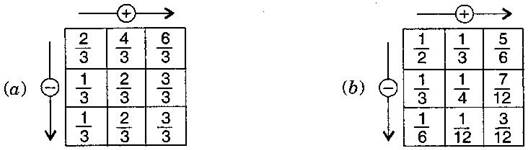###### Question 6.A piece of wire 7878 meter long broke into two pieces. One piece was 1414 meter long. How long is the other piece?

Total length of wire = 7878 meter

Length of first part = 1414 meter

Remaining part = 7814=7×12×1878–14=7×1–2×18 [ L.C.M. of 8 and 4 is 8]

728=587–28=58 meter

Therefore, the length of remaining part is 5858 meter.

NCERT Solutions for Class 6 Maths Exercise 7.6

###### Question 7.Nandini house is 910910 km from her school. She walked some distance and then took a bus for 1212 km to reach the school. How far did she walk?

Total distance between school and house = 910910 km

Distance covered by bus = 1212 km

Remaining distance = 91012=9×11×510910–12=9×1–1×510 [ L.C.M. of 10 and 2 is 10]

9510=410=259−510=410=25 km

Therefore, distance covered by walking us 2525 km.

###### Question 8.Ahsa and Samuel have bookshelves of the same size partly filled with books. Asha’s shelf is 56th56th full and Samuel’s shelf is 25th25th full. Whose bookshelf is more full? By what fraction?

5656 and 2525

56×55=253056×55=2530 and 25×66=123025×66=1230 [ L.C.M. of 6 and 5 is 30]

2530>12302530>1230  56>2556>25

Asha’s bookshelf is more covered than Samueal.

Difference = 25301230=13302530–1230=1330

NCERT Solutions for Class 6 Maths Exercise 7.6